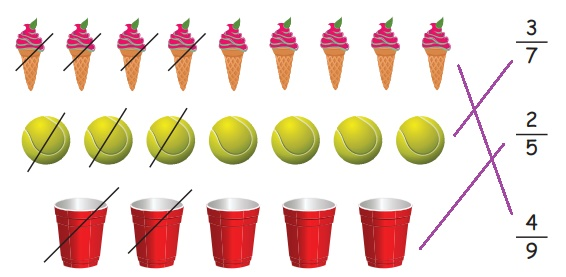Home | | Maths 4th Std | Exercise 6.2 (fractional part of a Collection)

# Exercise 6.2 (fractional part of a Collection)

Text Book Back Exercises Questions with Answers, Solution : 4th Maths : Term 2 Unit 6 : Fraction : Exercise 6.2 (fractional part of a Collection)

Exercise 6.2

1. Write the fractional part for the pictures that is circled.Match the following:Mark the given pictures with the fractions given below.Tags : Fraction | Term 2 Chapter 6 | 4th Maths , 4th Maths : Term 2 Unit 6 : Fraction
Study Material, Lecturing Notes, Assignment, Reference, Wiki description explanation, brief detail
4th Maths : Term 2 Unit 6 : Fraction : Exercise 6.2 (fractional part of a Collection) | Fraction | Term 2 Chapter 6 | 4th Maths